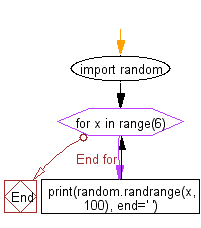﻿ Python Math: Generate random integers in a specific numerical range - w3resource# Python Math: Generate random integers in a specific numerical range

## Python Math: Exercise-49 with Solution

Write a Python program to generate random integers in a specific numerical range.

Sample Solution:-

Python Code:

``````import random

for x in range(6):
print(random.randrange(x, 100), end=' ')
```
```

Sample Output:

```21 55 48 50 27 5
```

Pictorial Presentation:Flowchart:## Visualize Python code execution:

The following tool visualize what the computer is doing step-by-step as it executes the said program:

Python Code Editor:

Have another way to solve this solution? Contribute your code (and comments) through Disqus.

What is the difficulty level of this exercise?

Test your Python skills with w3resource's quiz

﻿

## Python: Tips of the Day

Python: Get the Key Whose Value Is Maximal in a Dictionary

```>>> model_scores = {'model_a': 100, 'model_z': 198, 'model_t': 150}
>>> # workaround
>>> keys, values = list(model_scores.keys()), list(model_scores.values())
>>> keys[values.index(max(values))]
'model_z'
>>> # one-line
>>> max(model_scores, key=model_scores.get)
'model_z'
```